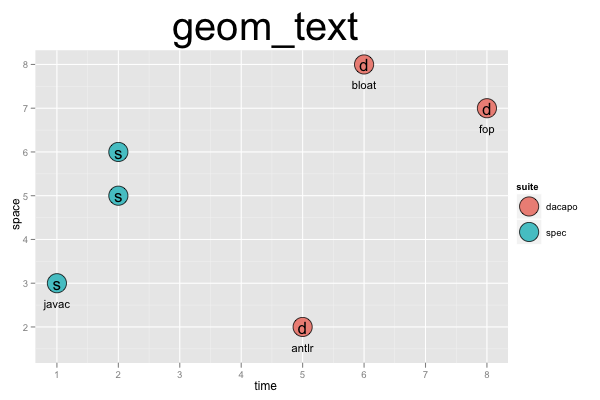## ggplot2 Quick Reference: geom_text

A geom that draws a text label at a given x and y coordinate.

Default statistic: stat_identity

### Parameters

• x - (required) x coordinate of the text label
• y - (required) y coordinate of the text label
• label - (required) the text for the label
• size - (default: 5) size of the font
• colour - (default: "black") the color of the text label
• alpha - (default: 1=opaque) the transparency of the text label
• hjust - (default: 0.5) position of the anchor (0=left edge, 1=right edge), can go below 0 or above 1
• vjust - (default: 0.5) position of the anchor (0=bottom edge, 1=top edge), can go below 0 or above 1
• angle - (default: 0=horizontal, left to right) the angle at which to draw the text label
• parse - (default: F)

### Example

This plot consists of three layers. First, we draw points. Then we draw single character labels centered over the points (the label corresponds to the first character of the "suite" column). Finally, we draw labels below the extreme points (the observations with the minimum and maximum "space" and "time", as determined by the call to `subset()`). To ensure that the labels below the extreme points are still visible, we explicitly define the y scale (`scale_y_continuous`) to manually specify its limits (`limits=c(1.5, 8)`).```d=data.frame(time=c(1,2,5,6,8,2), space=c(3,6,2,8,7,5), suite=c('spec','spec','dacapo','dacapo','dacapo','spec'), bm=c('javac','db', 'antlr', 'bloat', 'fop', 'raytrace'))
ex=subset(d, time<=min(time)|time>=max(time)|space<=min(space)|space>=max(space))
ggplot() +
scale_y_continuous(limits=c(1.5, 8)) +
geom_point(data=d, mapping=aes(x=time, y=space, fill=suite), size=9, shape=21, color="black") +
geom_text(data=d, mapping=aes(x=time, y=space, label=substr(suite, 1, 1)), size=6) +
geom_text(data=ex, mapping=aes(x=time, y=space, label=bm), size=4, vjust=3, hjust=0.5) +
opts(title="geom_text", plot.title=theme_text(size=40, vjust=1.5))```